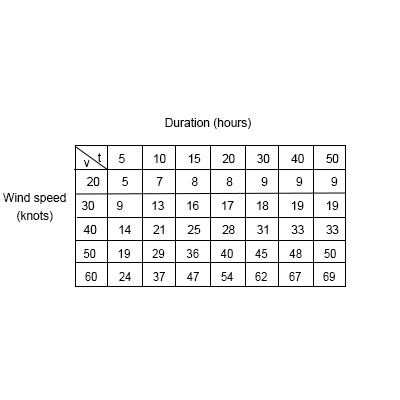# The wave heights h in the open sea depend on the speed v of the wind and the length of time t...

## Question:

The wave heights {eq}h {/eq} in the open sea depend on the speed {eq}v {/eq} of the wind and the length of time {eq}t {/eq} that the wind has been blowing at that speed. Values of the function {eq}h = f(v, t) {/eq} are recorded in feet in the following table.

a) Use the table to find a linear approximation to the wave height function when {eq}v {/eq} is near 40 knots and {eq}t {/eq} is near 20 hours. (Round your numerical coefficients to two decimal places.)

b) Estimate the wave heights when the wind has been blowing for 23 hours at 44 knots.## Linear Approximation:

The linear approximation or linearization of a function {eq}f(x,y) {/eq} at the point {eq}(a,b) {/eq}

can be found as the first order Taylor polynomial of the function at that point

{eq}\displaystyle L(x,y) = f(a,b) + f_x(a,b) (x-a) + f_y(a,b) (y-b). {/eq}

where {eq}f_x, \; f_y {/eq} are the first partial derivatives of the function.

We are given the values wave heights in the open sea as function of speed {eq}v {/eq} of wind and time {eq}t {/eq}

that is {eq}h = f(v, t) {/eq}.

a) The linear approximation of the wave height function when {eq}v = 40 {/eq} knots and {eq}t = 20 {/eq} hours

is equal to

{eq}\displaystyle L(v,t) = h(40,20) + h_v(40,20) (v-40) + h_t(40,20) (t-20). {/eq}

The partial derivatives are estimate from the data in the table as centered finite differences

{eq}\displaystyle h_v(40,20) = \frac{ h_v(50,20)- h(30,20) } { 20 } = \frac{ 40-17 } { 20 } = \frac{23}{20} \\ \displaystyle h_t(40,20) = \frac{ h_v(40,30)- h(40,15) } { 15 } = \frac{ 31-25 } { 15 } = \frac{2}{5} {/eq}

and so the linear approximation writes as

{eq}\displaystyle L(v,t) = 28 + \frac{23}{20} (v-40) + \frac{2}{5}(t-20) =\frac{23v}{20}+\frac{2t}{5}-26 \\ \displaystyle 1.15v +0.40t -26. {/eq}

b) The wave heights when the wind has been blowing for 23 hours at 44 knots is equal to

{eq}\displaystyle L(44,23) = 1.15(44) +0.40(23) -26 = 33.8 \; feet. {/eq}